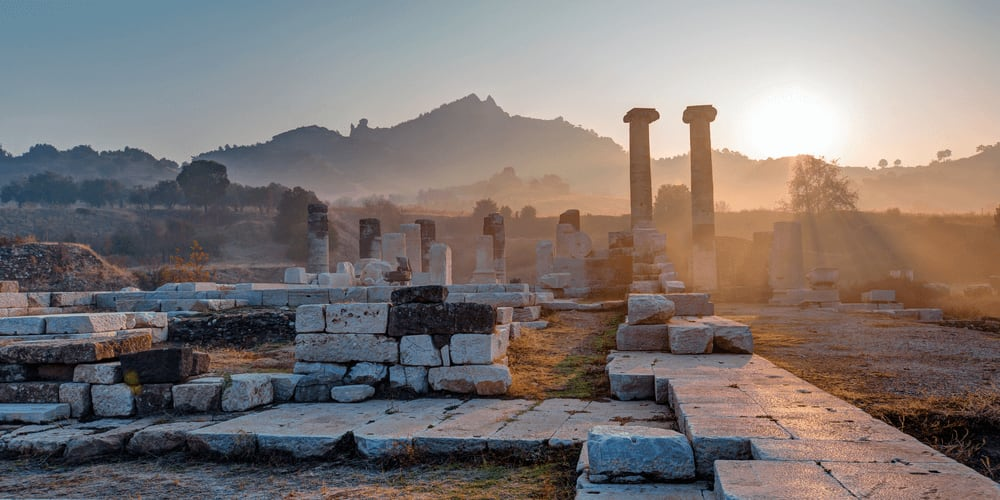# Greek Mathematics

Greek mathematics was mostly based on geometry; it’s therefore only natural that their most influential contributions to modern mathematics are related to geometryAncient Greece, a civilization that developed from about 12th-9th cc. BCE to 600 AD, occupied a large part of the Mediterranean region and Central Asia. At first, Greek mathematics was much influenced by mathematical practices of Ancient Egypt and Babylonia – for example, the ancient Greek numeral system resembled the Egyptian base 10 system. Like the Egyptians, the Greeks would use a symbol for “100” three times to represent the number “300.” However, the Ancient Greeks quickly moved beyond the mere adoption of certain elements to the development of their own practices. Since Greek mathematics was mostly based on geometry, it’s only natural that their most influential contributions to modern mathematics are related to geometry.

### ThalesThales of Miletus who is generally considered the founder of geometry not only understood similar and right triangles but also used his knowledge to find the height of pyramids.

### PythagorasPythagoras’s investigations marked the birth of Greek mathematics. Moreover, the very word “mathematics” is believed to be invented by Pythagoras! It derives from the Greek root “mathema” which means “knowledge” or “learning.” You can hardly find a person who has never heard of the Pythagorean theorem (a2+b2=c2) that is used for right-angled triangles. However, only a few people know that the ancient Greek mathematician didn’t invent the theorem – he was the first to prove it.

### PlatoAlthough Plato is mostly famous for his works in philosophy, he also made a great contribution to mathematics. He identified five regular symmetrical 3-dimensional shapes known as the Platonic Solids:

the tetrahedron that is composed of four regular triangles. According to Plato, the tetrahedron symbolized fire;

the octahedron that is made up of eight triangles. Plato said that octahedrons represented air;

the icosahedron that is constructed of 20 triangles. Plato the mathematician associated it with water;

the cube made up of 6 squares symbolized earth;

the dodecahedron that was constructed of 12 pentagons. Plato defined the figure as “the god used for arranging the constellations on the whole heaven.”

Quick quiz
Time to boost your math skills
Play Quiz